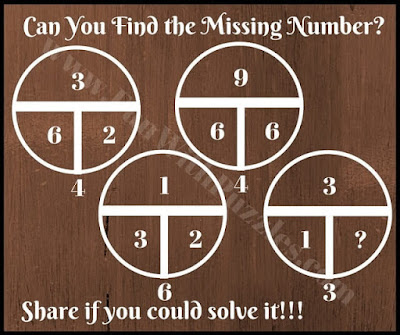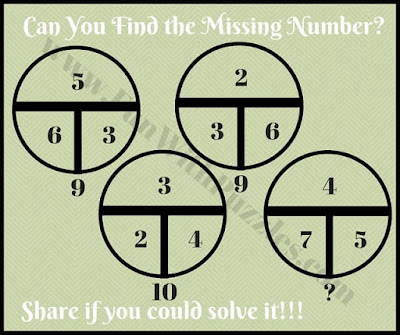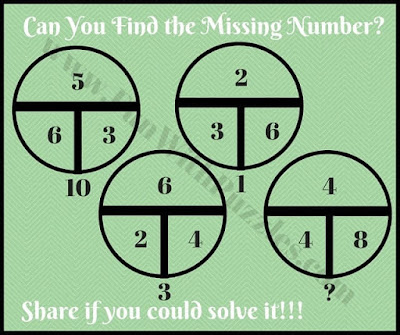For the last few months we have been posting the math circle puzzles and these puzzles are being loved by the visitors. So we are extending and posting more such Math Puzzles. These math puzzles are with the circles' picture. In fact, with the circle picture, there are many designs of similar type puzzles. Do check out other similar math puzzles.
In these circle math puzzles, one has to find the missing number in the circle. There are four circles with each circle having four numbers that share some logical or mathematical relationship with each other. Find this relationship and solve it for the missing number.
Answers to these puzzles will be provided later. However, do post your answers in the comment section at the end of this post.1. Can you find this missing number?2. Can you calculate the value of the missing number?3. What number replaces the question mark?4. Can you find this missing number value?5. Can you find the value of the missing number?

Also, do check out similar fun brain teasers and puzzles as mentioned below which will tickle your brain
List of Fun Brain Teasers and Puzzles

1. Fun Brain Teasers with Answers to Challenge your Mind: It contains mind-challenging fun brain teasers which are Mathematical, Logical as well as word brain teasers.

2. Mind Twisting Math Pattern Series Riddles with answers: These are Mathematical Mind Twisting pattern puzzles in which your challenge is to find the next number in the series.

3. Simple Math Riddles and Puzzles with Answers: It contains simple yet challenging Maths Riddles and Puzzles for Kids and Teens.

4. Elevate Mobile Training Games Review: If you have a smartphone, then you should try out this game on your mobile for sure which will help to enhance your Maths and English skills.

Answers of Math Circle Brain Teaser
Let's give the notations to the numbers in and around the Circle. Let A denote the upper half of the Circle. Let us say B and C to the lower half of the Circle from left to right and let's say D to the number below outside of the Circle.
1)
Logic used in this brain teaser is A x D = (B x 10) + C

2)
The logic used in this brain teaser is A x D = B x C

3)
The logic used in this brain teaser is A x B = C x D

4)